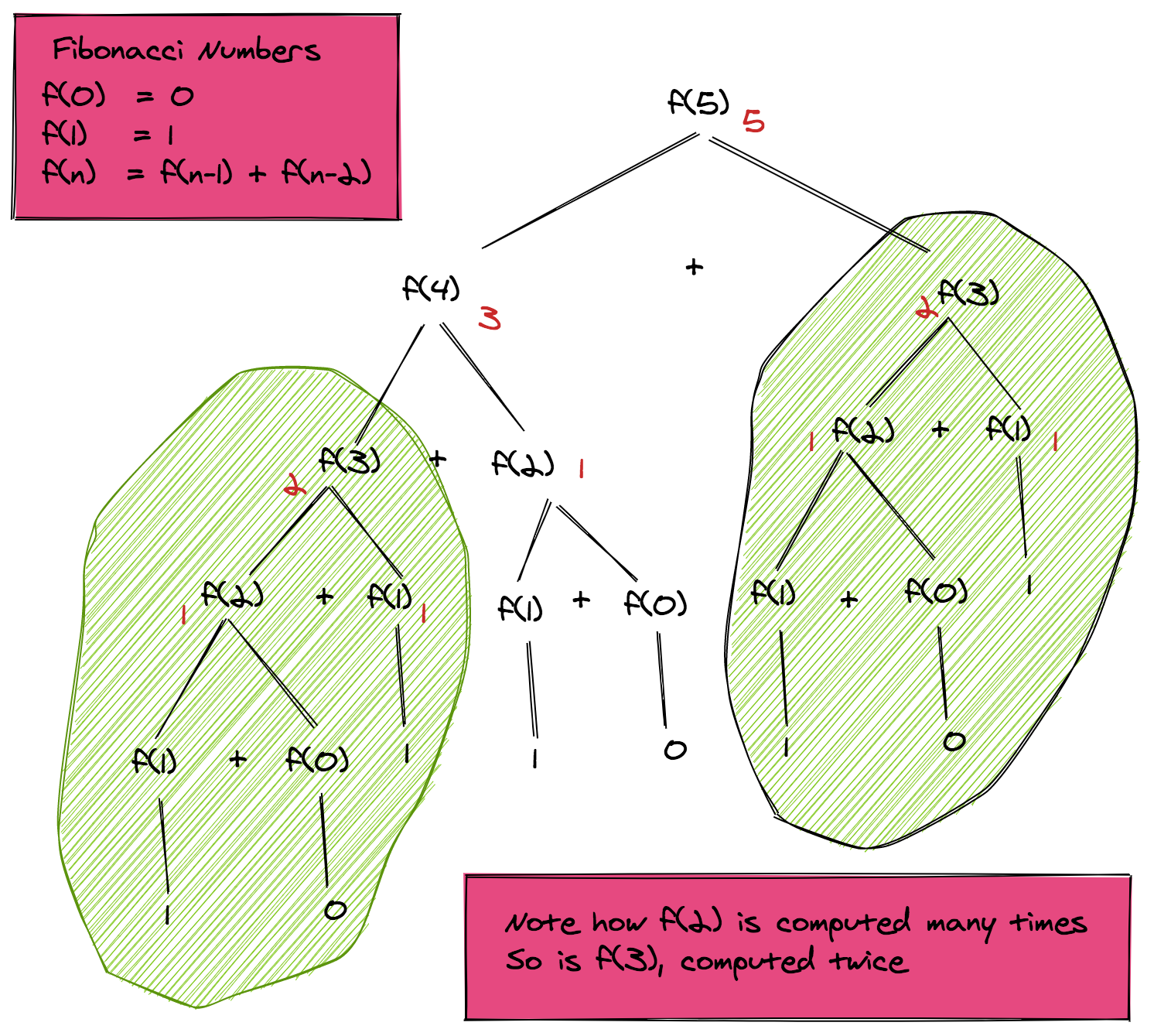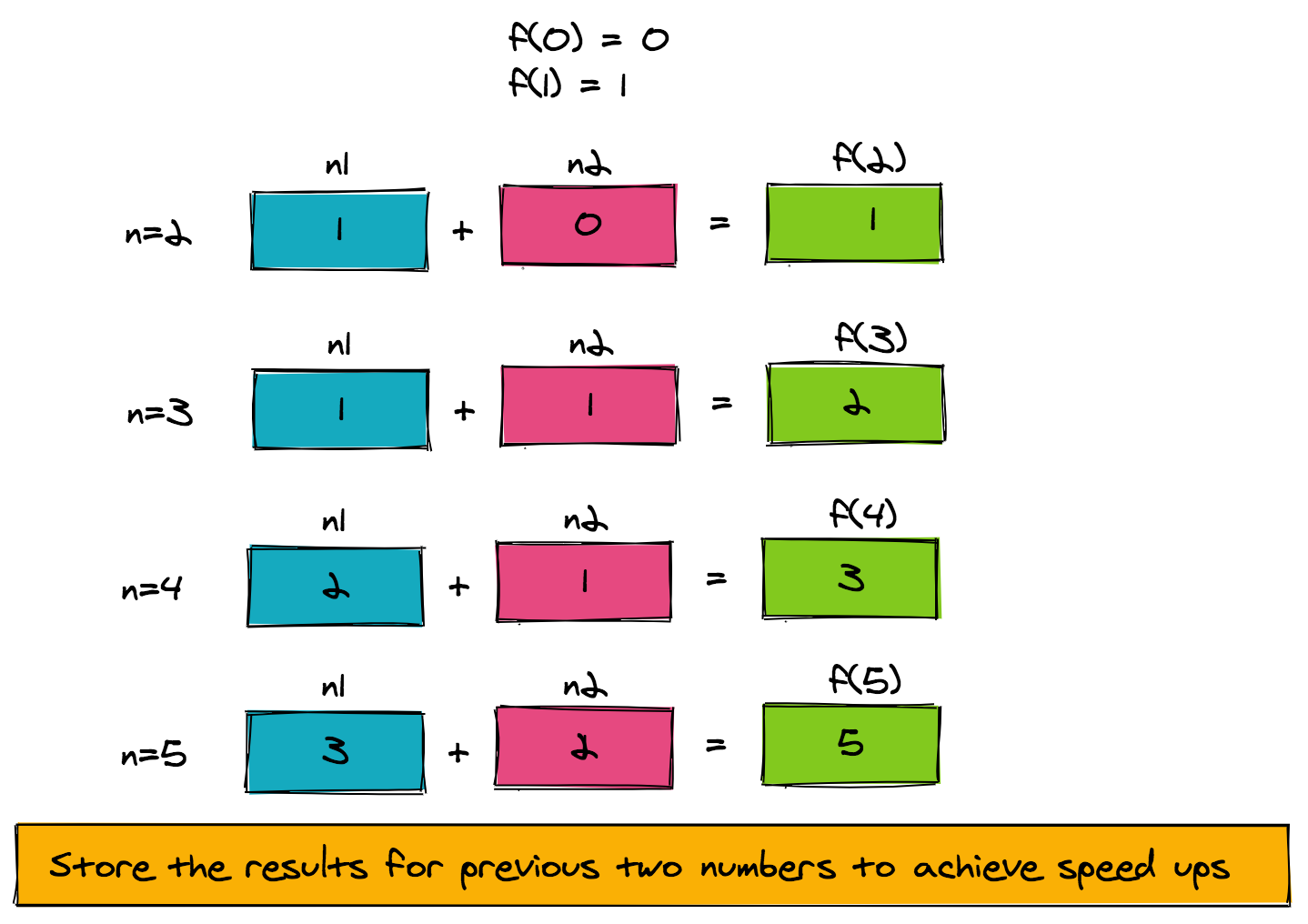1597352280

# Memoization in Dynamic Programming Through Examples

`Dynamic programming` is a technique for solving problems, whose solution can be expressed recursively in terms of solutions of overlapping sub-problems. A gentle introduction to this can be found in How Does DP Work? Dynamic Programming Tutorial.

This lesson was originally published at https://algodaily.com, where I maintain a technical interview course and write think-pieces for ambitious developers.

`Memoization` is an optimization process. In simple terms, we store the intermediate results of the solutions of sub-problems, allowing us to speed up the computation of the overall solution. The improvement can be reduced to an `exponential time solution to a polynomial time solution`, with an overhead of using additional memory for storing intermediate results.

Let’s understand how dynamic programming works with memoization with a simple example.## Fibonacci Numbers

You have probably heard of `[**Fibonacci numbers**](https://algodaily.com/challenges/fibonacci-sequence/)` several times in the past, especially regarding recurrence relations or writing recursive functions. Today we’ll see how this simple example gives us a true appreciation of the power of dynamic programming and memoization.

## Definition of Fibonacci Numbers

The nth Fibonacci number f(n) is defined as:

``````f(0) = 0                                       // base case
f(1) = 1                                       // base case
f(n) = f(n-1) + f(n-2)    for n>1              // recursive case
``````

The sequence of Fibonacci numbers generated from the above expressions is:

``````0 1 1 2 3 5 8 13 21 34 ...
``````

## Pseudo-code for Fibonacci Numbers

When implementing the mathematical expression given above, we can use the following recursive pseudo-code attached.

``````Routine: f(n)
Output: Fibonacci number at the nth place

Base case:
1\. if n==0 return 0
2\. if n==1 return 1
Recursive case:
1\. temp1 = f(n-1)
2\. temp2 = f(n-2)
3\. return temp1+temp2
``````

The recursion tree shown below illustrates how the routine works for computing `f(5)` or `fibonacci(5)`.

If we look closely at the recursive tree, we can see that the function is computed twice for `f(3)`, thrice for `f(2)` and many times for the base cases `f(1)` and `f(0)`. The overall complexity of this pseudo-code is therefore exponential O(2n). We can very well see how we can achieve massive speedups by storing the intermediate results and using them when needed.## Memoization of Fibonacci Numbers: From Exponential Time Complexity to Linear Time Complexity

To speed things up, let’s look at the structure of the problem. `f(n)` is computed from `f(n-1)` and `f(n-2)`. As such, we only need to store the intermediate result of the function computed for the previous two numbers. The pseudo-code to achieve this is provided here.

The figure below shows how the pseudo-code is working for `f(5)`. Notice how a very simple memoization technique that uses two extra memory slots has reduced our time complexity from exponential to linear (`**O(n)**`).``````Routine: fibonacciFast
Input: n
Output: Fibonacci number at the nth place
Intermediate storage: n1, n2 to store f(n-1) and f(n-2) respectively
1\. if (n==0) return 0
2\. if (n==1) return 1
3\. n1 = 1
4\. n2 = 0
5\. for 2 .. n
a. result = n1+n2           // gives f(n)
b.   n2 = n1                // set up f(n-2) for next number
c.   n1 = result            // set up f(n-1) for next number
6\. return result
``````

## Maximizing Rewards While Path Finding Through a Grid

Now that we understand `memoization` a little better, let’s move on to our next problem. Suppose we have an `m * n` grid, where each cell has a “reward” associated with it. Let’s also assume that there’s a robot placed at the starting location, and that it has to find its way to a “goal cell”. While it’s doing this, it will be judged by the path it chooses. We want to get to the “goal” via a path that collects the maximum reward. The only moves allowed are “up” or “right”.

#data-structures #computer-science #software-development #dynamic-programming #algorithms #algorithms

## Buddha Community1600398733

## All You Need to Know About Dynamic Programming

In this article, I will introduce the concept of dynamic programming, developed by Richard Bellman in the 1950s, a powerful algorithm design technique to solve problems by breaking them down into smaller problems, storing their solutions, and combining these to get to the solution of the original problem.

The hardest problems asked in FAANG coding interviews usually fall under this category. It is likely that you will get tasked with solving one during your interviews, hence the importance of knowing this technique. I will explain what dynamic programming is, give you a recipe to tackle dynamic programming problems, and will take you through a few examples so that you can understand better when and how to apply it.

#dynamic-programming #programming-interviews #programming

1624531051

Alerts4Dynamics is productivity app for Dynamics 365 CRM that helps to create, schedule, manage and track alerts. It helps to notify and pass relevant information to target audience right within Dynamics 365 CRM. These notifications can be created and displayed indefinitely or for a defined scheduled period. Notification button is available on all entities and can be accessed from anywhere in the CRM.
Features
• Create Announcement and Rule Based/Record Based alerts.
• Alerts can be sent as Pop-ups, Form Notifications or Email to target Dynamics 365 CRM users.
• Categorize alerts as Information, Warning or Critical.
• Define process start date from when the notifications will start getting created and process end date when creation of new notifications will stop. Also, add the display end date for notification.

1625057623

# Undo changes & restore records in Dynamics 365 CRM with a single click

Click2Undo is a productivity app that helps you to undo changes in the data in Dynamics 365 CRM with a single click. Be it the last change that you’d want to restore, or the changes that were done in the past which you would like to get back, Click2Undo can do it without any hassle. This provides a safety net within which users can conduct day-to-day activities without fear of losing data due to human or technical errors.
Click2Undo is available for Dynamics CRM 8.2 and above, Dataverse (Power Apps). It supports deployment models - On-Premises and Online.
Features
• Entity Support: Click2Undo provides support to all OOB as well as Custom Entities
• Undo Last Changes: Ability to restore the last changes done to a Dynamics 365 CRM record by clicking the Click2Undo button
• Undo Past Changes: Ability to undo past changes made to multiple fields on Dynamics 365 CRM records in one go using History button
• Undo Bulk Changes: Ability to undo changes on multiple records at one go.

#restore last state of dynamics 365 records #restoring deleted dynamics 365 records #recovering deleted dynamics 365 records #recover deleted dynamics crm records #dynamics 365 online recover deleted records #restore records dynamics crm1597352280

## Memoization in Dynamic Programming Through Examples

`Dynamic programming` is a technique for solving problems, whose solution can be expressed recursively in terms of solutions of overlapping sub-problems. A gentle introduction to this can be found in How Does DP Work? Dynamic Programming Tutorial.

This lesson was originally published at https://algodaily.com, where I maintain a technical interview course and write think-pieces for ambitious developers.

`Memoization` is an optimization process. In simple terms, we store the intermediate results of the solutions of sub-problems, allowing us to speed up the computation of the overall solution. The improvement can be reduced to an `exponential time solution to a polynomial time solution`, with an overhead of using additional memory for storing intermediate results.

Let’s understand how dynamic programming works with memoization with a simple example.## Fibonacci Numbers

You have probably heard of `[**Fibonacci numbers**](https://algodaily.com/challenges/fibonacci-sequence/)` several times in the past, especially regarding recurrence relations or writing recursive functions. Today we’ll see how this simple example gives us a true appreciation of the power of dynamic programming and memoization.

## Definition of Fibonacci Numbers

The nth Fibonacci number f(n) is defined as:

``````f(0) = 0                                       // base case
f(1) = 1                                       // base case
f(n) = f(n-1) + f(n-2)    for n>1              // recursive case
``````

The sequence of Fibonacci numbers generated from the above expressions is:

``````0 1 1 2 3 5 8 13 21 34 ...
``````

## Pseudo-code for Fibonacci Numbers

When implementing the mathematical expression given above, we can use the following recursive pseudo-code attached.

``````Routine: f(n)
Output: Fibonacci number at the nth place

Base case:
1\. if n==0 return 0
2\. if n==1 return 1
Recursive case:
1\. temp1 = f(n-1)
2\. temp2 = f(n-2)
3\. return temp1+temp2
``````

The recursion tree shown below illustrates how the routine works for computing `f(5)` or `fibonacci(5)`.

If we look closely at the recursive tree, we can see that the function is computed twice for `f(3)`, thrice for `f(2)` and many times for the base cases `f(1)` and `f(0)`. The overall complexity of this pseudo-code is therefore exponential O(2n). We can very well see how we can achieve massive speedups by storing the intermediate results and using them when needed.## Memoization of Fibonacci Numbers: From Exponential Time Complexity to Linear Time Complexity

To speed things up, let’s look at the structure of the problem. `f(n)` is computed from `f(n-1)` and `f(n-2)`. As such, we only need to store the intermediate result of the function computed for the previous two numbers. The pseudo-code to achieve this is provided here.

The figure below shows how the pseudo-code is working for `f(5)`. Notice how a very simple memoization technique that uses two extra memory slots has reduced our time complexity from exponential to linear (`**O(n)**`).``````Routine: fibonacciFast
Input: n
Output: Fibonacci number at the nth place
Intermediate storage: n1, n2 to store f(n-1) and f(n-2) respectively
1\. if (n==0) return 0
2\. if (n==1) return 1
3\. n1 = 1
4\. n2 = 0
5\. for 2 .. n
a. result = n1+n2           // gives f(n)
b.   n2 = n1                // set up f(n-2) for next number
c.   n1 = result            // set up f(n-1) for next number
6\. return result
``````

## Maximizing Rewards While Path Finding Through a Grid

Now that we understand `memoization` a little better, let’s move on to our next problem. Suppose we have an `m * n` grid, where each cell has a “reward” associated with it. Let’s also assume that there’s a robot placed at the starting location, and that it has to find its way to a “goal cell”. While it’s doing this, it will be judged by the path it chooses. We want to get to the “goal” via a path that collects the maximum reward. The only moves allowed are “up” or “right”.

#data-structures #computer-science #software-development #dynamic-programming #algorithms #algorithms1598532420

## An Introduction to Dynamic Programming

Although people make a big deal about how scary dynamic programming problems are, there’s really no need to be afraid of them. In fact, once you get the hang of them, these can actually be very easy problems.

What is dynamic programming?

_Dynamic programming is a technique to solve problems by breaking it down into a collection of sub-problems, solving each of those sub-problems just once and storing these solutions inside the cache memory in case the same problem occurs the next time. Dynamic Programming is mainly an optimization over plain _recursion . Wherever we see a recursive solution that has repeated calls for same inputs, we can optimize it using Dynamic Programming. This simple optimization reduces the time complexities from exponential to polynomial.

Briefly speaking, dynamic programming is mostly just a matter of taking a recursive algorithm and finding the overlapping subproblems (that is, the repeated calls). You then cache those results for future recursive calls. Alternatively, you can study the pattern of the recursive calls and implement something iterative. You still “cache” previous work.

There are two different ways to store our values so that they can be reused. Let’s discuss both of them:

1. Tabulation or the Bottom Up approach.

2. Memoization or the Top Down Approach.Tabulation vs Memoization

A note on terminology: Some people call top-down dynamic programming “memoization” and only use “dynamic programming” to refer to bottom-up work.

#dynamic-programming #computer-science #software-development #programing #big-o-notation #big data